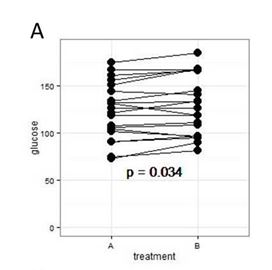## how to do a dot plot for paired samples

Hi,

How do I do a plot that looks like this?

Any sample code would be highly appreciated.

YiFan

simple dot plot with connecting lines for paired samples:1 ACCEPTED SOLUTION

Accepted Solutions

## Re: how to do a dot plot for paired samples

If you have a variable which identifies each pair then something like

proc sgplot data=have noautolegend nocycleattrs;

series x=treatment y=glucose / group=Pairidvariable markers Markerattrs= (symbol=CircleFilled)

;

run;

4 REPLIES 4

## Re: how to do a dot plot for paired samples

If you have a variable which identifies each pair then something like

proc sgplot data=have noautolegend nocycleattrs;

series x=treatment y=glucose / group=Pairidvariable markers Markerattrs= (symbol=CircleFilled)

;

run;

tyvm 🙂

## Re: how to do a dot plot for paired samples

Here's one way using SGPLOT. The key thing is to get your data in the right format.

``````data have;
call streaminit(20);

do i=1 to 10;
glucose=rand('normal', 120, 10); A=10; output;
glucose=rand('normal', 120, 10); A=30;
output;
end;
run;

proc sgplot data=have;
series x=A y=glucose/group=i markers MARKERATTRS=(Symbol=circlefilled);
xaxis values=(0 to 40 by 10);
run;``````

## Re: how to do a dot plot for paired samples

tyvm 🙂

Discussion stats
• 4 replies
• 2856 views
• 0 likes
• 3 in conversation